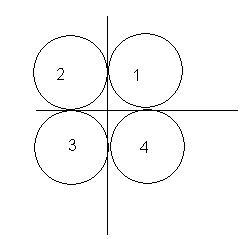# 3-d collision

4 identical balls of radius R and mass m are lying in a gravity free space. The balls are in contact and their centres are forming vertices of a square of side 2R in horizontal plane. One identical ball travelling vertically wid speed v hits the 4 balls symmetrically.. The collision is perfectly elastic.Q1. wat is the speed of ball 1 after collision??
Q2. What is the speed of the ball which was initially travelling wid v?
Q3. Unit vector in dir of motion of ball 2 is wat?

•Lokesh Verma ·

there was a similar question of the day which i thought was a unique question..

where did u get this from?

•Lokesh Verma ·

http://targetiit.com/iit_jee_forum/posts/19th_november_2008_587.html

•Shreyan ·

this question was dere in fiitjee open test

•Ankit Shukla ·

Consider the system of 4 balls to be acting on the COM.

Momentum conservation equation along z-axis:---

mv = 4m*v1 - m*v2 ---- (i)

As, the travelling ball will return by the same path due to symmmetry.

e = velocity of separation/velocity of approach

1 = (v1+v2)/v

v = v1+v2 ---- (ii)

Solving 1 & 2

we get,

v2 = 3v/5;

Now apply energy conservation equation before and after collision, as it is elastic collision

energy will be conserved.

consider, speed of 4 similar balls = v3

0.5mv^2 = 0.5*4m*(v3)^2 + 0.5 *m*((3/5)v)^2

m*(16/25)*v^2 = 4m*(v3)^2

v3 = 2v/5;

•